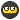# How to calc distance between 2 lat/long points?

## Recommended Posts

Hi, if I have 2 lat/long pairs, how do I determine the distance between the 2 points in feet?

I would prefer a spreadsheet but a Win XP program would be fine too.

Hi, if I have 2 lat/long pairs, how do I determine the distance between the 2 points in feet?

I would prefer a spreadsheet but a Win XP program would be fine too.

Use the great circle distance between two points. Two good references are wikipedia and the whizzo bang aviation formulary

In Java, for example:

double d = Math.acos(Math.sin(lat1) * Math.sin(lat2) + Math.cos(lat1) * Math.cos(lat2) * Math.cos(lon1 - lon2));

Where the units are in radians; Real men don't use feet-- There's an obscure application called Google that you can use to find out how to convert to other units but, generally, you pretend to be at the equator (approximately 6378137 metres, captain).

Edit: I just noticed that the aviation formulary page actually does have an Excel spreadsheet..

Edited by poohstickz

Use fizzymagic's GeoCalc program

Plot them on a map and use the scale at the bottom.

If you don't think they are too far from each other and you have a mapping GPSr, input both sets of coordinates into it. Then go to the map page and measure the distance between them.

Since you are asking for the calculations in feet, I assume that you are talking short distances, less than two miles. A set of equations that you could use for a spherical approximation follow:

L1 = Latitude of Point 1

λ1 = Latitude of Point 1

L2 = Latitude of Point 2

λ2 = Latitude of Point 2

The distance north/south in feet will be:

ΔL = 364566*( L1 - L2 )

The distance east/west in feet will be:

Δλ = 364566*( λ1 - λ2 )*cos(( L1 + L2 )/2)

Using the Pythagorean Theorem the distance may now be calculated as:

Dist = SQRT(ΔL*ΔL + Δλ*Δλ)

Notes:

1. Coordinates should be expressed in decimal degree format.

2. This method will give you fairly accurate results on distances up to 10 miles.

3. These calculations should not be used if you are caching in extreme polar coordinates, like latitudes above 87º.

4. Despite what you may read in these forums no system of distance calculation is completely accurate, surveyors have compared them to actual measured conditions. There are some methods of calculation that the error is less than one part in ten thousand.

5. If you understand what I wrote above, it should be no problem putting it on a spreadsheet. However, it will take time. Be careful on using the correct format on all angles.

6. You may find it easier if you use UTM coordinates. Although accuracy using these coordinates drops as you approach a different zone (at Longitudes of -72º, -78º, -84º, -90º, -96º,…..). It would also be inaccurate in extreme polar coordinates.

send me an email and i'll send you an excel spreadsheet that calculates direction and distance between two lats and longs. jeffreyfort@mac.com

## Join the conversation

You can post now and register later. If you have an account, sign in now to post with your account.
Note: Your post will require moderator approval before it will be visible.×   Pasted as rich text.   Paste as plain text instead

Only 75 emoji are allowed.